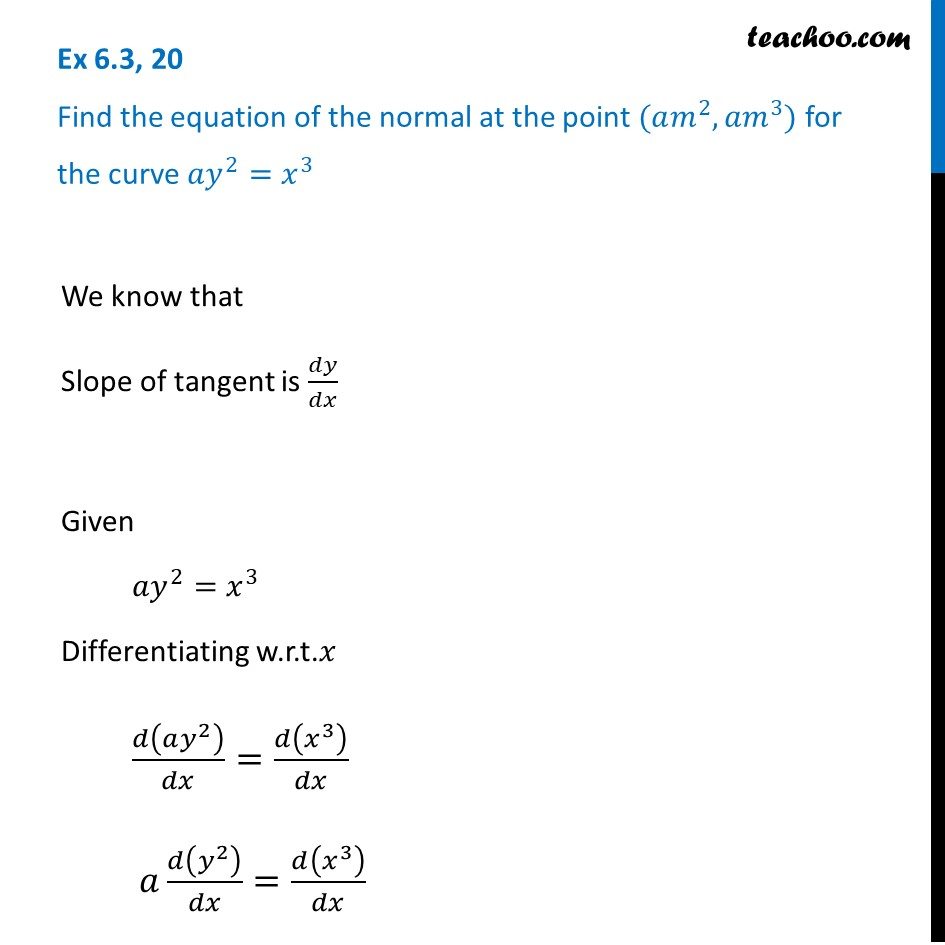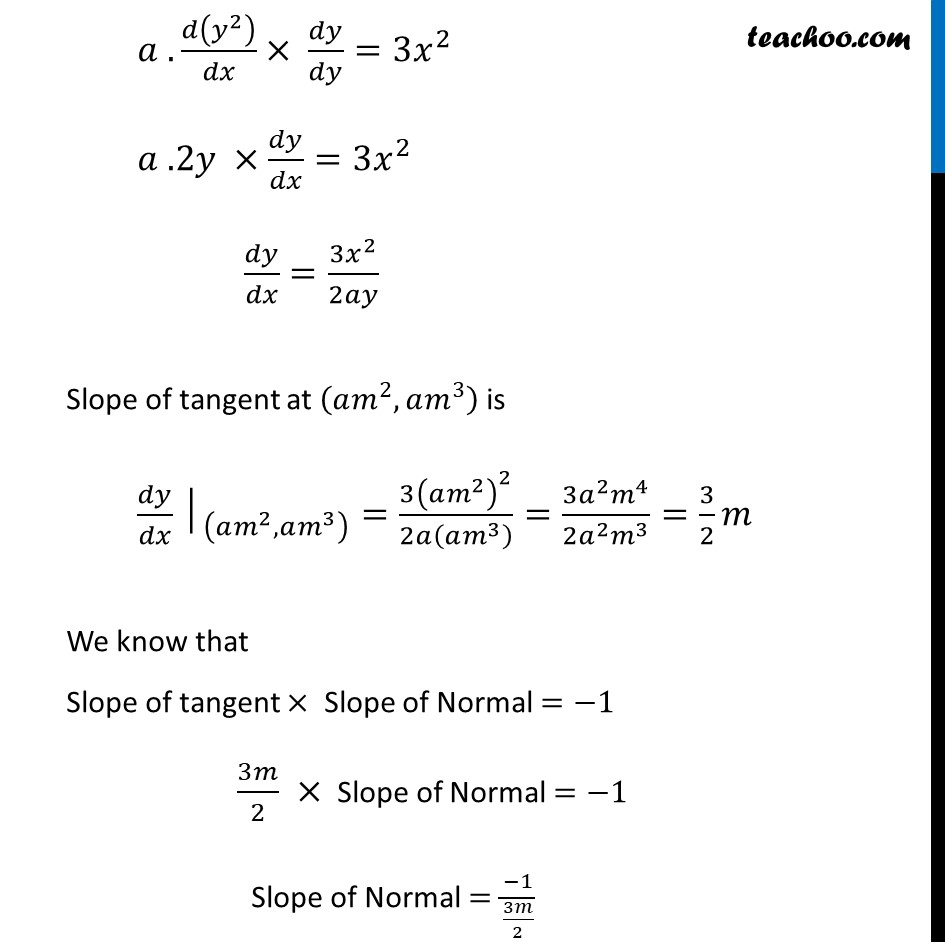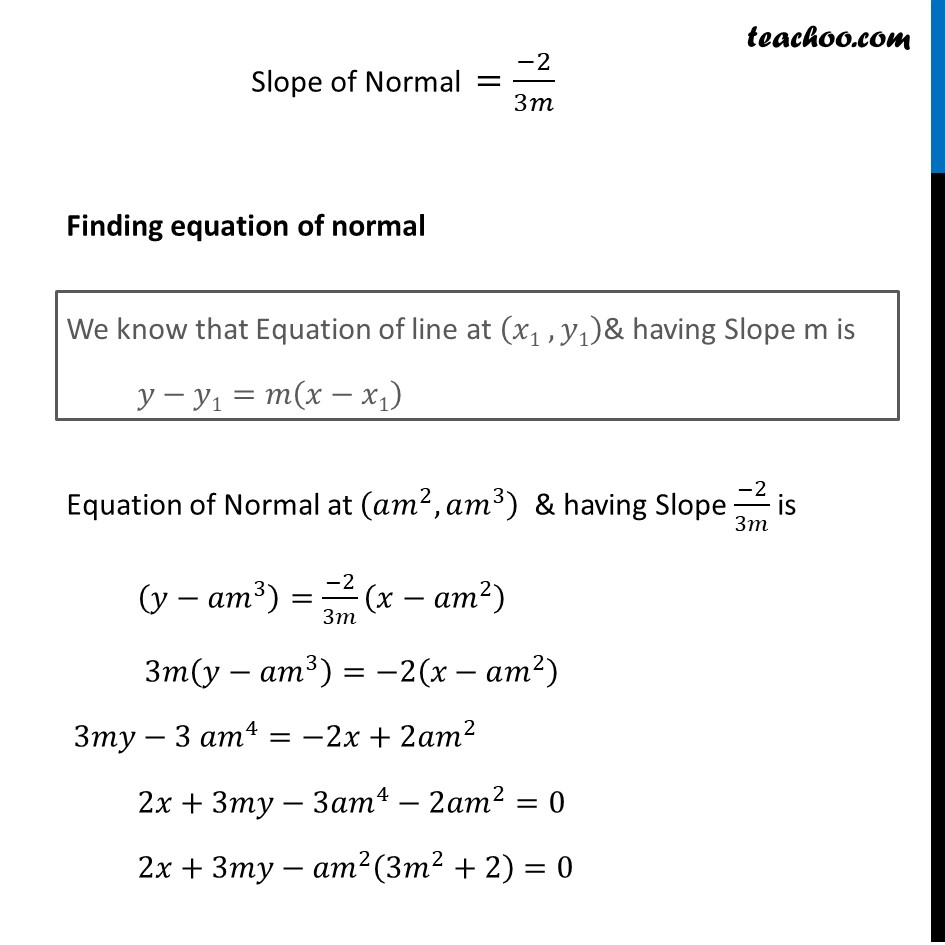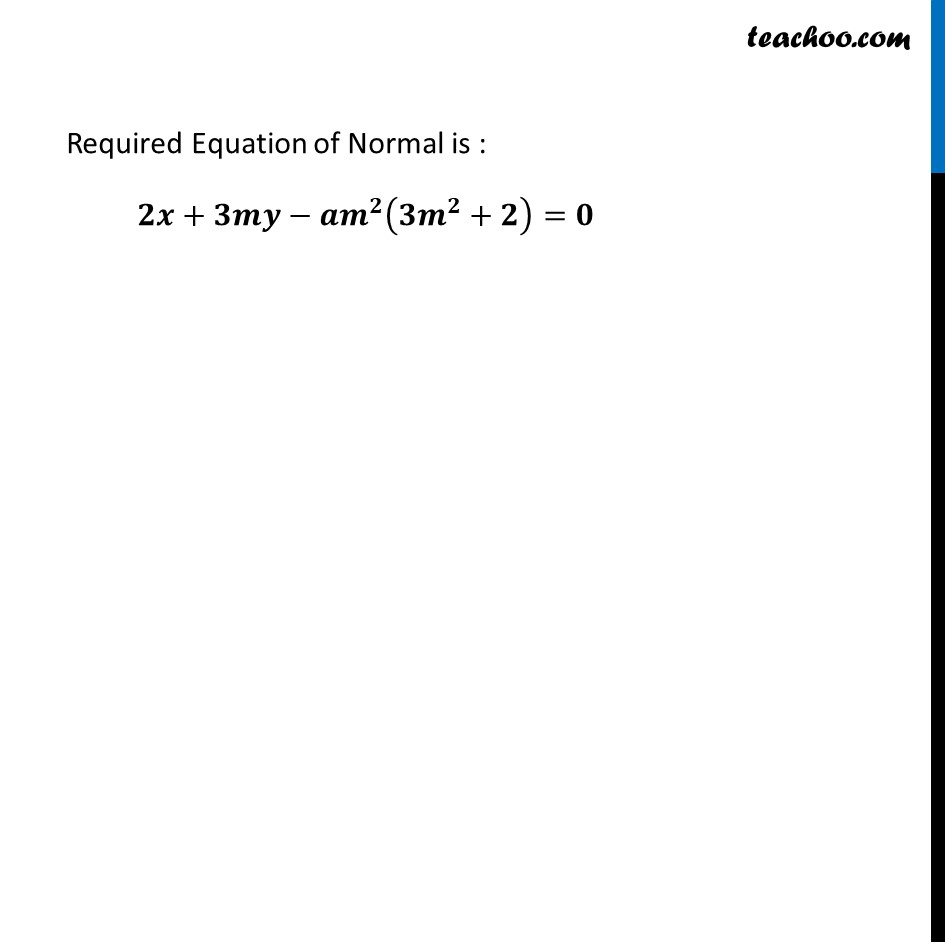Finding equation of tangent/normal when point and curve is given

Chapter 6 Class 12 Application of Derivatives
Concept wiseLearn in your speed, with individual attention - Teachoo Maths 1-on-1 Class

### Transcript

Question 20 Find the equation of the normal at the point (𝑎𝑚^2,𝑎𝑚^3) for the curve 𝑎𝑦^2=𝑥^3We know that Slope of tangent is 𝑑𝑦/𝑑𝑥 Given 𝑎𝑦^2=𝑥^3 Differentiating w.r.t.𝑥 𝑑(𝑎𝑦^2 )/𝑑𝑥=𝑑(𝑥^3 )/𝑑𝑥 𝑎 𝑑(𝑦^2 )/𝑑𝑥=𝑑(𝑥^3 )/𝑑𝑥 𝑎 . 𝑑(𝑦^2 )/𝑑𝑥× 𝑑𝑦/𝑑𝑦=3𝑥^2 𝑎 .2𝑦 ×𝑑𝑦/𝑑𝑥=3𝑥^2 𝑑𝑦/𝑑𝑥=(3𝑥^2)/2𝑎𝑦 Slope of tangent at (𝑎𝑚^2,𝑎𝑚^3 ) is 〖𝑑𝑦/𝑑𝑥│〗_((𝑎𝑚^2,𝑎𝑚^3 ) )=(3(𝑎𝑚^2 )^2)/2𝑎(𝑎𝑚^3 ) =(3𝑎^2 𝑚^4)/(2𝑎^2 𝑚^3 )=3/2 𝑚 We know that Slope of tangent × Slope of Normal =−1 3𝑚/2 × Slope of Normal =−1 Slope of Normal =(−1)/(3𝑚/2) Slope of Normal =(−2)/3𝑚 Finding equation of normal Equation of Normal at (𝑎𝑚^2, 𝑎𝑚^3 ) & having Slope (−2)/3𝑚 is (𝑦−𝑎𝑚^3 )=(−2)/3𝑚 (𝑥−𝑎𝑚^2 ) 3𝑚(𝑦−𝑎𝑚^3 )=−2(𝑥−𝑎𝑚^2 ) 3𝑚𝑦−3 𝑎𝑚^4=−2𝑥+2𝑎𝑚^2 2𝑥+3𝑚𝑦−3𝑎𝑚^4−2𝑎𝑚^2=0 2𝑥+3𝑚𝑦−𝑎𝑚^2 (3𝑚^2+2)=0 We know that Equation of line at (𝑥1 , 𝑦1)& having Slope m is 𝑦−𝑦1=𝑚(𝑥−𝑥1) Required Equation of Normal is : 𝟐𝒙+𝟑𝒎𝒚−𝒂𝒎^𝟐 (𝟑𝒎^𝟐+𝟐)=𝟎